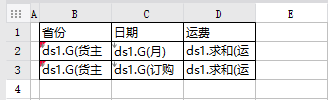# 根据年份、月份分别进行月份和日期汇总## 1. 描述编辑## 4. 实现方案编辑

4.1 新建数据集

ds1：
SELECT 订购日期,strftime('%Y',订购日期) as 年,strftime('%m',订购日期) as 月 ,运货费,货主省份 FROM s订单 where 1=1 \${if(len(a)=0,'',"and strftime('%Y',订购日期) in ('"+a+"')")} \${if(len(b)=0,'',"and strftime('%m',订购日期) in ('"+b+"')")} order by 订购日期
ds2：作为年控件数据字典
SELECT strftime('%Y',订购日期) as 年 FROM s订单 order by 订购日期
ds3：作为月控件数据字典
SELECT strftime('%m',订购日期) as 月 FROM s订单 where 1=1 \${if(len(a)=0,'',"and strftime('%Y',订购日期) in ('"+a+"')")} order by 订购日期
4.2 模板设计4.3 添加条件属性## 5. 效果预览编辑

5.1 PC端预览效果

5.2 移动端预览效果
app效果：h5效果：